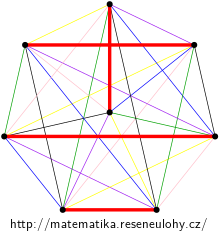## Chromatic index of complete graph

Determine the chromatic index, i.e. the edge chromatic number, of the complete graph $$K_n$$.

• #### Hint

Distinguish the cases of even and odd $$n$$.

• #### Solution

For even $$n$$, draw the vertices of the graph into the vertices of a regular $$(n-1)$$ -gon and place the last vertex in its center. Any edge leading from center to the boundary can be extended to a perfect matching so that the added edges are perpendicular to the starting edge.

These matchings are disjoint to each other and prove that $$\chi’(K_n) = \Delta (K_n) = n-1$$, see figure.For odd $$n$$ we notice that in each maximum matching, one vertex remains uncovered. Therefore, each maximum matching has $$\frac{n-1}{2}$$ edges. The graph $$K_n$$ has a total of $$\frac{n (n-1)}{2}$$ edges, so each edge coloring must have at least $$n$$ classes.

Since $$K_n$$ is a subgraph of $$K_{n + 1}$$, we get $$\chi'(K_n) \leq \chi' (K_{n + 1}) = n$$.

The chromatic index of the complete graph $$K_n$$ is $$\chi (K_n) = \begin{cases} n-1 & n \text{ is even} \\ n & n \text{ is odd} \end{cases}$$.